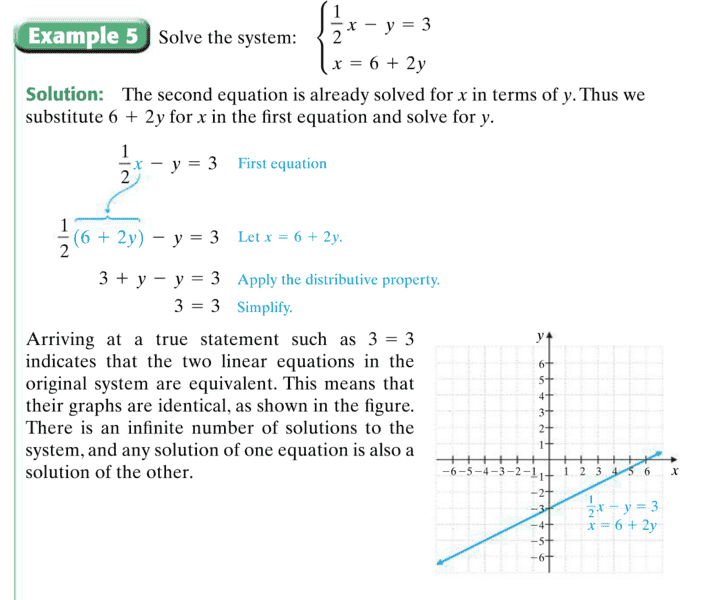# Solving Systems of Linear Equations in Two Variables- Graphs

DS2C

## Homework Statement

Solve the system of equations: { (1/2)x-y=3 and x=6+2y

NA

## The Attempt at a Solution

The solution is 3=3, which is an identity, which means that there is an infinite amount of solutions to the system. Here's where my question lies (asked my teacher but she didn't know):
This system of equations results in two graphs, or lines. Their graphs are identical, so on a graph it would look like a single line. But are they two different lines occupying the same space on the plane, or are they the same line? I hope this makes sense. I've attached a screenshot of the problem out of the book for reference.Ray Vickson
Homework Helper
Dearly Missed

## Homework Statement

Solve the system of equations: { (1/2)x-y=3 and x=6+2y

NA

## The Attempt at a Solution

The solution is 3=3, which is an identity, which means that there is an infinite amount of solutions to the system. Here's where my question lies (asked my teacher but she didn't know):
This system of equations results in two graphs, or lines. Their graphs are identical, so on a graph it would look like a single line. But are they two different lines occupying the same space on the plane, or are they the same line? I hope this makes sense. I've attached a screenshot of the problem out of the book for reference.View attachment 216102

There is only one line. Any point (x,y) that satisfies one of the equations automatically also satisfies the other.

•DS2C
kuruman
Homework Helper
Gold Member
The two equations are not linearly independent which is another way of saying that one can be obtained from the other and there is only one straight line. Just multiply the bottom equation by 1/2 and move y to the left and you will see what I mean.

•DS2C
DS2C
So theyre not two lines occupying the same space. They are one line that resulted from two different equations. Or are they the same equation just in different forms since multiplying by 1/2 turns it into the top one?

Mark44
Mentor
So theyre not two lines occupying the same space. They are one line that resulted from two different equations. Or are they the same equation just in different forms since multiplying by 1/2 turns it into the top one?
It's really only one line. The two equations are equivalent, meaning that any solutions (ordered pairs (x, y)) of one equation are also solutions of the other equation.

•SammyS and DS2C
DS2C
Ok thank you guys. Cleared that up.

scottdave
•